Question

# Consider this reaction: 2NaOH(aq) + H2(g) →2Na(s) + 2H2O(l)                     Δ H°sys = +367.58 kJ/mol    ΔS°sys = –11.40 J/K·mo

Consider this reaction:
2NaOH(aq) + H2(g) →2Na(s) + 2H2O(l)                     Δ H°sys = +367.58 kJ/mol    ΔS°sys = –11.40 J/K·mol

Calculate  ΔSuniv for this reaction at 25 °C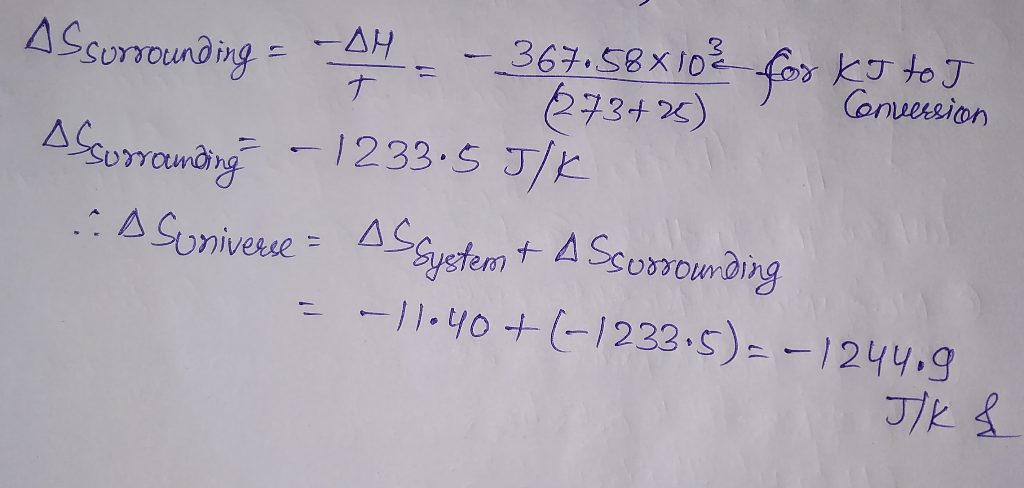#### Earn Coins

Coins can be redeemed for fabulous gifts.

Similar Homework Help Questions
• ### For the following reaction: 2Na(s) + 2H2O(l) → 2NaOH(s) + H2(g) Compound ΔH°f (kJ mol-1) S°...

For the following reaction: 2Na(s) + 2H2O(l) → 2NaOH(s) + H2(g) Compound ΔH°f (kJ mol-1) S° (J mol-1 K-1) Na (s) 0.00 51.30 H2O (l) -285.83 69.91 NaOH (s) -425.93 64.46 H2 (g) 0.00 130.68 Calculate ΔG°rx (in kJ) at 391.96 K for this reaction.  Assume ΔH°f and S° do not vary as a function of temperature.

• ### For the reaction 2Na(s) + 2H2O(1) -2NaOH(aq) + H2(g) AH° -368.6 kJ and AS --15.3 J/K...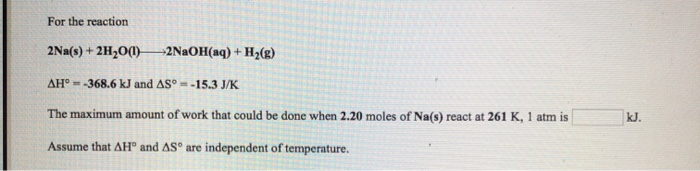For the reaction 2Na(s) + 2H2O(1) -2NaOH(aq) + H2(g) AH° -368.6 kJ and AS --15.3 J/K The maximum amount of work that could be done when 2.20 moles of Na(s) react at 261 K, 1 atm is kJ. Assume that AH and AS are independent of temperature.

• ### Enter your answer in the provided box. Consider the reaction 2Na(s) + 2H2O(1) 2NaOH(aq) + H2)...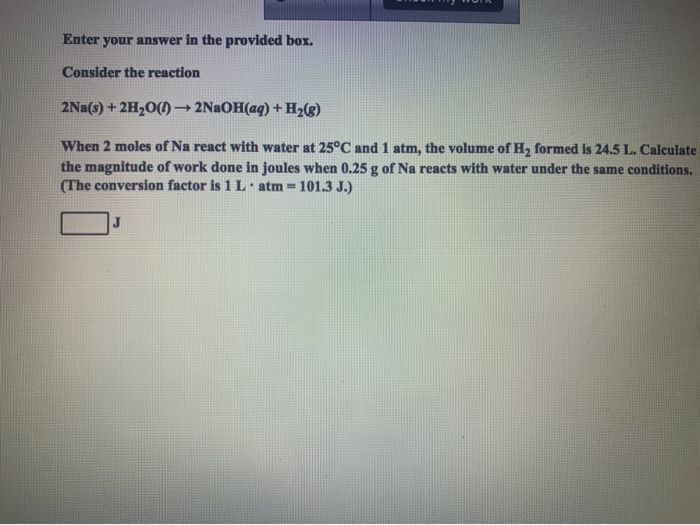Enter your answer in the provided box. Consider the reaction 2Na(s) + 2H2O(1) 2NaOH(aq) + H2) When 2 moles of Na react with water at 25°C and 1 atm, the volume of H2 formed is 24.5 L. Calculate the magnitude of work done in joules when 0.25 g of Na reacts with water under the same conditions. (The conversion factor is 1 L atm = 101.3 J.) J

• ### Sodium reacts with water according to the reaction: 2Na(s)+2H2O(l)→2NaOH(aq)+H2(g) Identify the oxidizing agent. a) H2O(l) b)...

Sodium reacts with water according to the reaction: 2Na(s)+2H2O(l)→2NaOH(aq)+H2(g) Identify the oxidizing agent. a) H2O(l) b) NaOH(aq) c) Na(s) d) H2 (g)

• ### The reaction of sodium(s) with water(l) to form sodium hydroxide(aq) and hydrogen(g) proceeds as follows: 2Na(s)...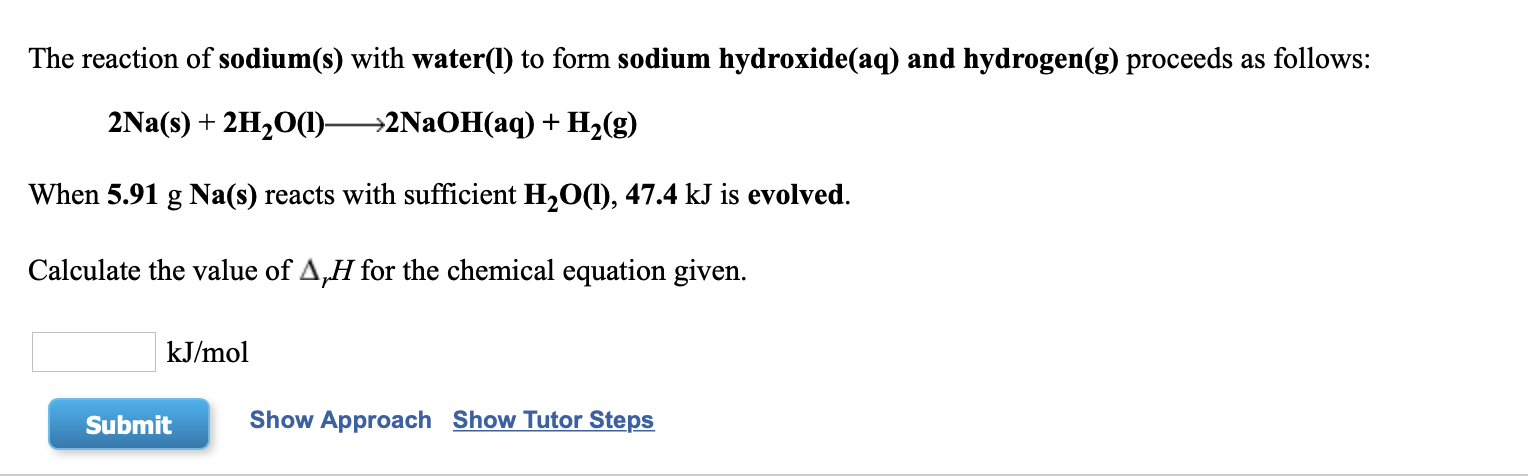The reaction of sodium(s) with water(l) to form sodium hydroxide(aq) and hydrogen(g) proceeds as follows: 2Na(s) + 2H2O(1)—>2NaOH(aq) + H2(g) When 5.91 g Na(s) reacts with sufficient H2O(1), 47.4 kJ is evolved. Calculate the value of A,H for the chemical equation given. kJ/mol Submit Show Approach Show Tutor Steps

• ### For the reaction Fe(s) + 2HCI(aq)FeCl2(s) + H2(g) ΔΗο--7.4 kJ and ΔS°-107.9 J/K The standard free...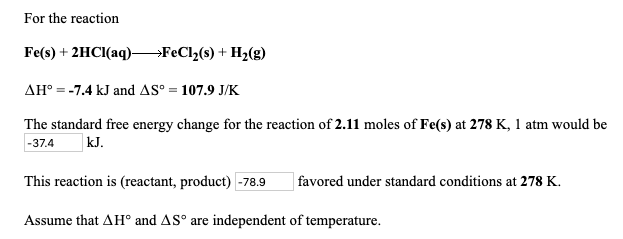For the reaction Fe(s) + 2HCI(aq)FeCl2(s) + H2(g) ΔΗο--7.4 kJ and ΔS°-107.9 J/K The standard free energy change for the reaction of 2.11 moles of Fe(s) at 278 K, 1 atm would be -37.4kJ This reaction is (reactant, product) -78.9 favored under standard conditions at 278 K Assume that Δ Ho and Δ are independent of temperature. For the reaction N2(g) + O2(g)2 NO(g) Δσ 172.7 kJ and ΔS°-24.9 J/K at 318 K and 1 atm. This reaction is (reactant,...

• ### Calculate ΔG°rxn for the reaction shown (DO NOT INCLUDE UNITS IN YOUR ANSWER) 2Na(s) + 2H2O(l)...

Calculate ΔG°rxn for the reaction shown (DO NOT INCLUDE UNITS IN YOUR ANSWER) 2Na(s) + 2H2O(l) --> 2NaOH (aq) + H2 (g) Calculate ΔG°rxn for the reaction shown  (DO NOT INCLUDE UNITS IN YOUR ANSWER) Ti(s) + 2Cl2 (g) --> TiCl4 (l)

• ### 41. Which reaction can be predicted from the activity series? a. 2H2O(aq) + 2Na(s) 2NaOH(aq) +...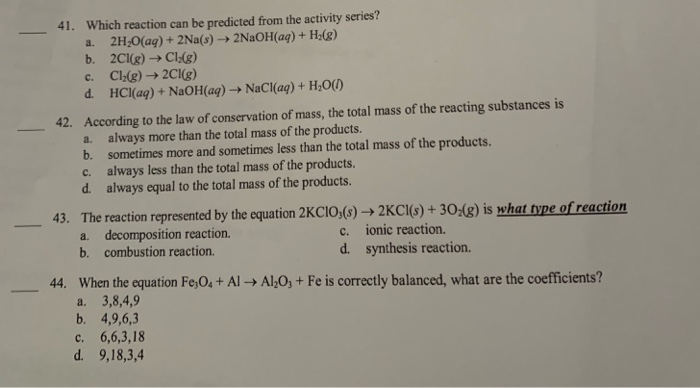41. Which reaction can be predicted from the activity series? a. 2H2O(aq) + 2Na(s) 2NaOH(aq) + H2(g) b. 2Cl(g) Cl:(8) c. Cl:(g) + 2Cl(g) d. HCl(aq) + NaOH(aq) NaCl(aq) + H2O(1) 42. According to the law of conservation of mass, the total mass of the reacting substances is a. always more than the total mass of the products. b. sometimes more and sometimes less than the total mass of the products. c. always less than the total mass of the...

• ### Consider the following reaction at 298 K. C(graphite)+2H2(g)⟶CH4(g)ΔH∘=−74.6 kJ and ΔS∘=−80.8 J/KC(graphite)+2H2(g)⟶CH4(g)ΔH∘=−74.6 kJ and ΔS∘=−80.8 J/K...

Consider the following reaction at 298 K. C(graphite)+2H2(g)⟶CH4(g)ΔH∘=−74.6 kJ and ΔS∘=−80.8 J/KC(graphite)+2H2(g)⟶CH4(g)ΔH∘=−74.6 kJ and ΔS∘=−80.8 J/K Calculate the following quantities. ΔSsys=ΔSsys J/K ΔSsurr= J/K ΔSuniv= J/K

• ### For each of the following reactions, predict the sign of ΔS°. 2Na(s) + Cl2(g) → 2NaCl(s)...

For each of the following reactions, predict the sign of ΔS°. 2Na(s) + Cl2(g) → 2NaCl(s) KBr(s) → K+(aq) + Br−(aq) 2H2(g) + O2(g) → 2H2O(l) C2H2(g) + 4F2(g) → 2CF4(g) + H2(g)